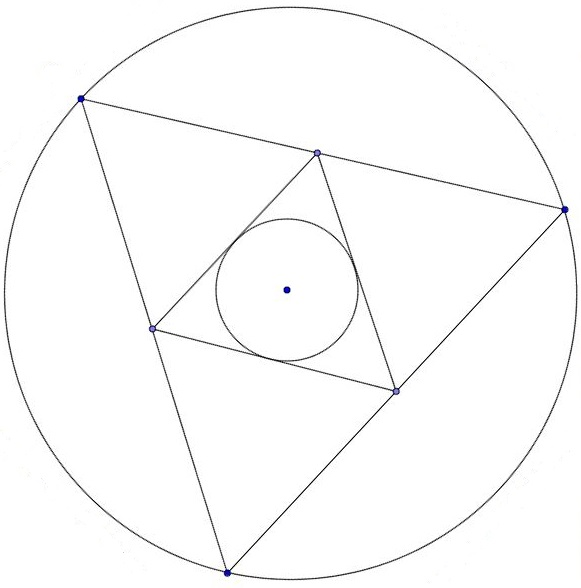# It's staring at meLet $\Delta ABC$ be a triangle with vertices $A=(0,0), B=(6,0), C=\big(3,3\sqrt{3}\big),$ and $\Delta PQR$ the pedal triangle of $\Delta ABC.$

The sum of the circumradius of $\Delta ABC$ and inradius of $\Delta PQR$ can be expressed as $\frac{a\sqrt{b}}{c}$ for positive integers $a,b,$ and $c,$ where $a,c$ are coprime and $b$ is square-free.

Find $a\times b\times c$.

×